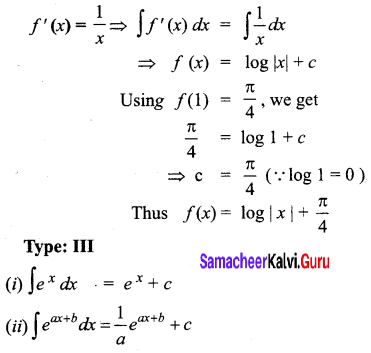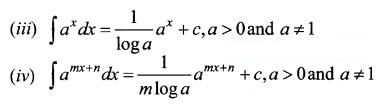# Samacheer Kalvi 12th Business Maths Solutions Chapter 2 Integral Calculus I Ex 2.2

Students can download 12th Business Maths Chapter 2 Integral Calculus I Ex 2.2 Questions and Answers, Samacheer Kalvi 12th Business Maths Book Solutions Guide Pdf helps you to revise the complete Tamilnadu State Board New Syllabus and score more marks in your examinations.

## Tamilnadu Samacheer Kalvi 12th Business Maths Solutions Chapter 2 Integral Calculus I Ex 2.2

Integrate the following with respect to x.

Question 1.
$$\int\left(\sqrt{2 x}-\frac{1}{\sqrt{2 x}}\right)^{2}$$
Solution: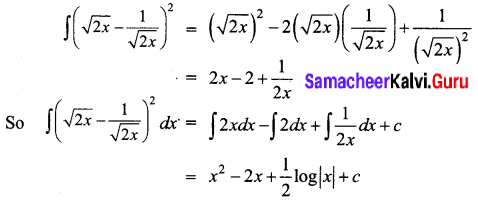Question 2.
$$\frac{x^{4}-x^{2}+2}{x-1}$$
Solution: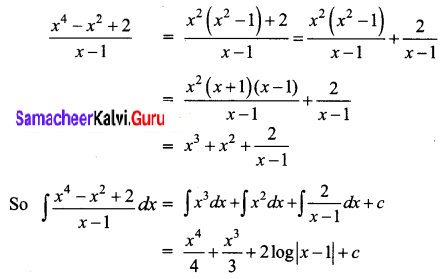Question 3.
$$\frac{x^{3}}{x+2}$$
Solution: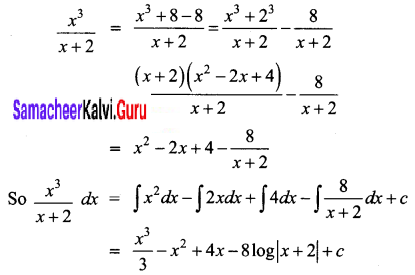Question 4.
$$\frac{x^{3}+3 x^{2}-7 x+11}{x+5}$$
Solution:
We have to find the quotient by division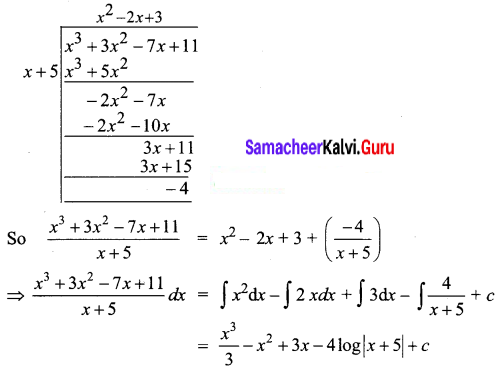Question 5.
$$\frac{3 x+2}{(x-2)(x-3)}$$
Solution:
We use partial fraction method to split the given function into two fractions and then integrate.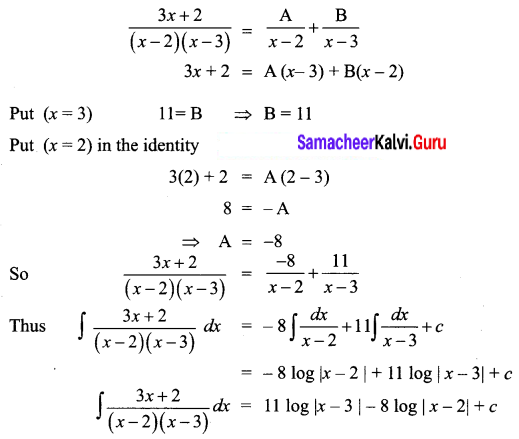Question 6.
$$\frac{4 x^{2}+2 x+6}{(x+1)^{2}(x-3)}$$
Solution:
By partial fractions,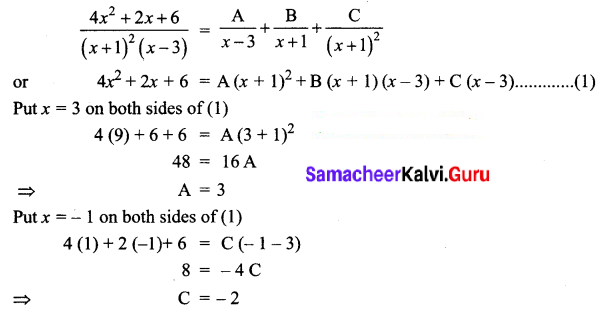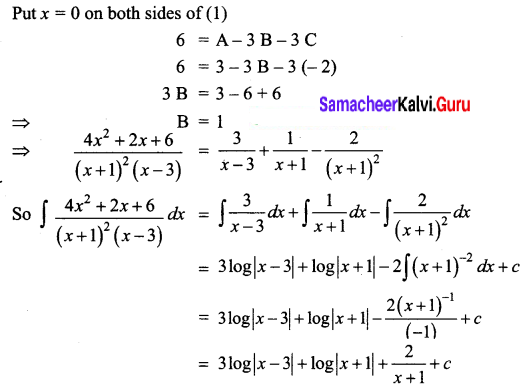Question 7.
$$\frac{3 x^{2}-2 x+5}{(x-1)\left(x^{2}+5\right)}$$
Solution: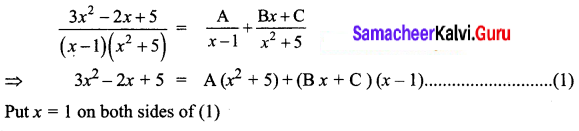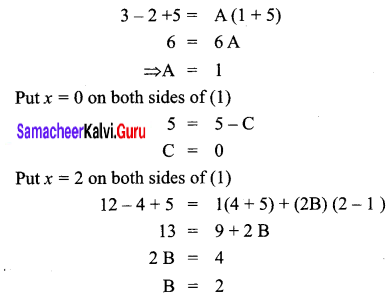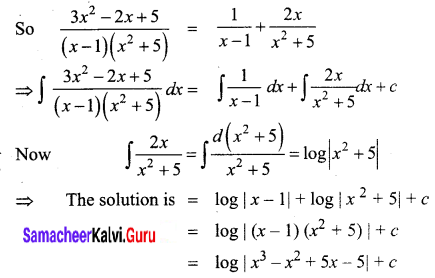Question 8.
If f'(x) = $$\frac{1}{x}$$ and f(1) = $$\frac{\pi}{4}$$, then find f(x)
Solution: